Pointers are generally regarded as somewhat confusing to learn in C. This is because they introduce not just new syntax, but a new way of thinking. Despite this initial difficulty, they are extremely useful and commonly used.

This article discusses pointers from a C perspective. However, pointers in C++ are effectively identical.

To understand pointers, we must first understand pointers. Wait, that is recursion. I mean to say, to understand pointers we first need to understand variables.

As you know, variables are used like this:

```int number = 7;

char letter = 'a';```

Those variables aren’t magic. They are stored somewhere in memory. We can picture memory as a series of boxes: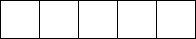Variables represent these boxes in memory. For example, number can be the first box. When we say “number = 7,” it means to put 7 into the number box.

After we create the number variable, memory looks something like this: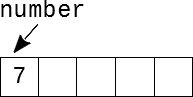All well and good so far. When we use number in the code it refers to that box. We can either put new things in the box or take a look at what is in there already.

These boxes have addresses as well, so we can tell them apart. It looks like this: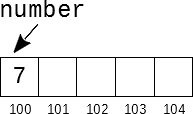The number box has address 100. Putting something in the number box is the same as putting something in box 100. The boxes for other variables will be located at other memory addresses.

Memory smemory, you say. Show me the pointers!

I was just getting to that. It is rude to interrupt, you know. Kids these days. Anyway.

Pointers are variables. Like variables, pointers have boxes. For normal variables, we put a value – say, 7 – into the box. For pointers, we put a memory address into the box instead. Suppose we have this code:

```int number = 7;
int * pointy = &number;```

There is some new syntax here. The * means it is a pointer – an int pointer, in this case. Pointers are a certain type, much like how char and int are types. The & means “address of.” It gets the memory address of the number box and stores it in pointy the pointer’s box.

Now memory looks like this: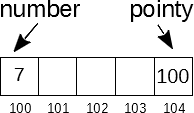Number hasn’t changed. Pointy now holds the address of the number box. They are called pointers because their value “points” to another variable – in this case, number.

Pointers effectively refer to a box in memory. The memory address uniquely identifies that box, so we can interact with its contents just like when we use a variable. We can get the value in the box pointed to as well as modify what is there:

```int number = 7;
int * pointy = &number;
*pointy = 8; //number = 8
int number2 = *pointy; //number2 = 8```

The * symbol has another meaning. If you use the * symbol next to a pointer variable after creating it, it dereferences the pointer. Dereferencing a pointer makes it act as if it is the thing it points to. In this example, pointy points to number. When we dereference pointy, it acts like it is number. As a result, we set number to 8. Similarly, the bottom expression becomes equivalent to “number2 = number1.”

Note that pointers can only point to a specific type. Int pointers point to ints, char pointers point to chars, and so on. They cannot be mixed, like a char pointer to an int.

That is more or less how pointers work. They have numerous applications.

## The -> Operator

Let’s say we have a struct:

```typedef struct
{
int x;
int y;
} Point;```

We can have a pointer to this type, and use it as expected:

```Point point = {25, 60};
Point * pointer = &point;

int x = (*pointer).x; //25```

To get the value of x, we must first dereference the pointer. We then access the x variable from the resulting point. The parenthesis are necessary due to precedence. The . operator has higher precedence than the * operator, so without parenthesis it would end up being *(pointer.x), which isn’t what we want.

This syntax is cumbersome. Fortunately, the language designers noted this and made a shortcut: ->. When you use -> on a pointer, it dereferences the pointer and accesses whatever comes next. This lets us do this:

`int x = pointer->x;`

## Null Pointers

Most of the time, pointers are pointing at something. That is why they are called pointers, you know.

Sometimes they aren’t pointing at anything, though. Much more polite that way, don’t you think?

Why aren’t they pointing at something? The reasons vary, most of which will make sense when you use pointers frequently. Maybe the object they will point to isn’t known yet, or perhaps the object it was pointing to is no longer of use and you don’t want to point to it anymore. In any case, null pointers are common.

When we do want a pointer to point to nothing, we set it to 0. Really:

`int * pointy = 0;`

Pointy is now a null pointer. This is also a good thing to initialize pointers to when they aren’t immediately assigned an address. As with other variables, pointers start with junk if left uninitialized.

Null pointers are primarily used to check if there is something “on the other end.” Due to the way C’s if conditionals work (anything nonzero is true), pointers in conditionals are convenient to use:

```int * pointy = 0;

//later

if (pointy)
printf("%d", *pointy);```

If pointy is null, then it is like saying “if (0),” and 0 is false. If pointy is pointing to something – say, it holds the memory address 500016 – then the conditional is true, and we print out the value at that address.

Note that you cannot dereference a null pointer. If you do, your program will crash.

## Void Pointers

Void pointers. Talk about a cool name.

Anyway, they are a specific type of pointer. They have the unique property of being able to point to anything. Earlier I mentioned that you cannot point a pointer of type A to a value of type B. That is true. However, you can point a void pointer to any type of value. For example:

```int number = 6;
char letter = 'a';
long john = 277705;

void * pointy;

pointy = &number;
pointy = &letter;
pointy = &john;```

Magic.

There is a caveat, though. You cannot dereference a void pointer. This is because the compiler wouldn’t know what type it would be. If you tried something like:

`voidPointer->maybeThisExists;`

The compiler has no way of checking if that type has a variable called “maybeThisExists.”

In order to get the value from a void pointer, you have to assign it (or cast it) to a pointer of the correct type. Note that no cast is needed to assign a void pointer to a pointer of another type.

```int number = 5;
void * voidPointer = &number;
int * intPointer = voidPointer;
printf("%d", *intPointer); //5```

It is important to make sure you use the correct pointer type when getting the value back. If the types are mismatched, the program will not work correctly and will likely crash. There is no way to find out the type of a void pointer, you must know its type from the context (e.g. you know what was put in there from earlier).

What are void pointers used for? A prime example is malloc. Malloc gives you newly allocated memory, but it doesn’t know what type you want so it returns a void pointer instead. Most other uses are somewhat advanced and wouldn’t make much sense without using them.

Also, while void pointers do exist in C++, they are effectively never used as better alternatives exist.

## Arrays

As it turns out, pointers and arrays are very closely related. Suppose we have this array:

`int array[] = {1, 2, 3, 4, 5};`

In memory, this gives us: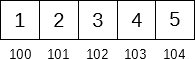I feel like counting. We should count up every number in this array and see what we get.

```int array[] = {1, 2, 3, 4, 5};
int sum = 0;

for (int i = 0; i < 5; ++i)
sum += array[i];```

That works. What if I need to count multiple arrays? I could rewrite this again, but it would be better to make a function that does it for me. Simple enough, but how do we pass the array to the function? We use a pointer.

When you pass an array to a function, it decays into a pointer to its first element. We can make our function like this:

```int sumArray(int * array, int size)
{
int sum = 0;

for (int i = 0; i < size; ++i)
sum += array[i];

return sum;
}

//somewhere else

int array[] = {1, 2, 3, 4, 5};

int sum = sumArray(array, 5);```

The array argument in sumArray is a pointer to the first element of the array – it points to box 100.

What happens with array[i]? Array[i] is equivalent to *(array + i). For example, array is *(array + o), which is *array, which evaluates to the first element of the array. Array is then *(array + 1), which will grab the next element. You do have to pass in the size of the array as well – there is no way to find the size from the array argument by itself.

Weird C trick side-note: array[i] is also equivalent to i[array]. This makes sense when you consider the other equivalence, as it yields *(i + array), and addition is commutative.

## Strings

Pointers are also closely related to strings. Suppose we have the string “Hello World.” Memory looks like this: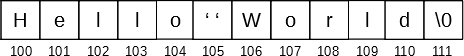As before, when you pass or assign a string to something, it turns into a pointer to the first element – the first character ‘H’, in this case.  If you inspect the argument types of functions that take strings – printf, for example – you will find their first argument is char *.

As an example, here is how you can find the length of a string:

```int strlen(char * str)
{
int length = 0;

while (*str != '\0')
{
++length;

++str;
}

return length;
}

//later

int i = strlen("Hello World"); //11```

Finding the length of a string is simple. We simply count each character until we reach the null terminator – if we found that, we are at the end of the string. In the while loop, we have *str. Str is a char *, so dereferencing it gives us the character it points to. The first time the loop runs, it is pointing to ‘H’. When the loop body runs, we increment the pointer – this makes it point to the next address, which is also the next character. By doing this we go through the string until we get to the end.

Passing strings is convenient due to their properties. You can pass in the string literal itself or a pointer to its first character. Thus, we only pass a pointer – often the same size as an int – whether our string is ten characters or ten million characters. Unlike arrays, we don’t need to pass in the length (though we could) because the length can be found by making something like the strlen function – or using the actual strlen function in the standard library.

## One thought on “C Pointers”

1. Todd Ojala says:

Very nice explanation of one of the more confusing aspects of C (to newbies).